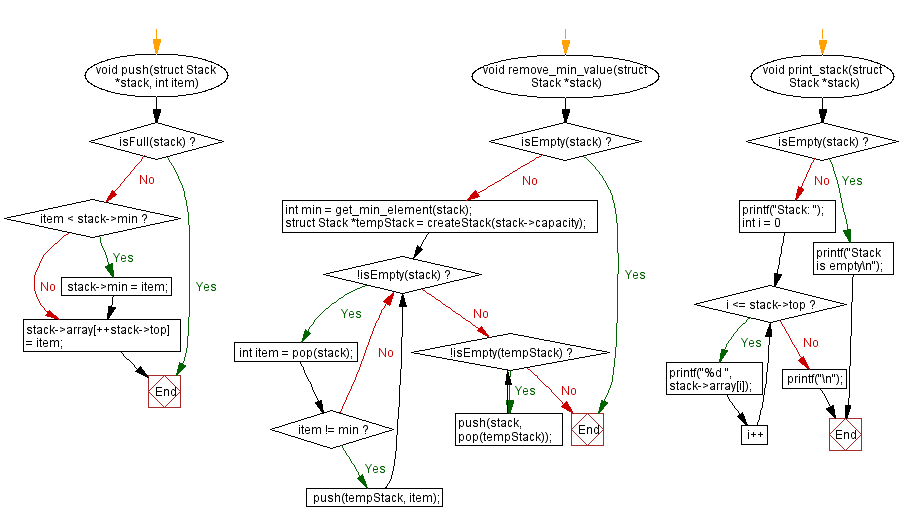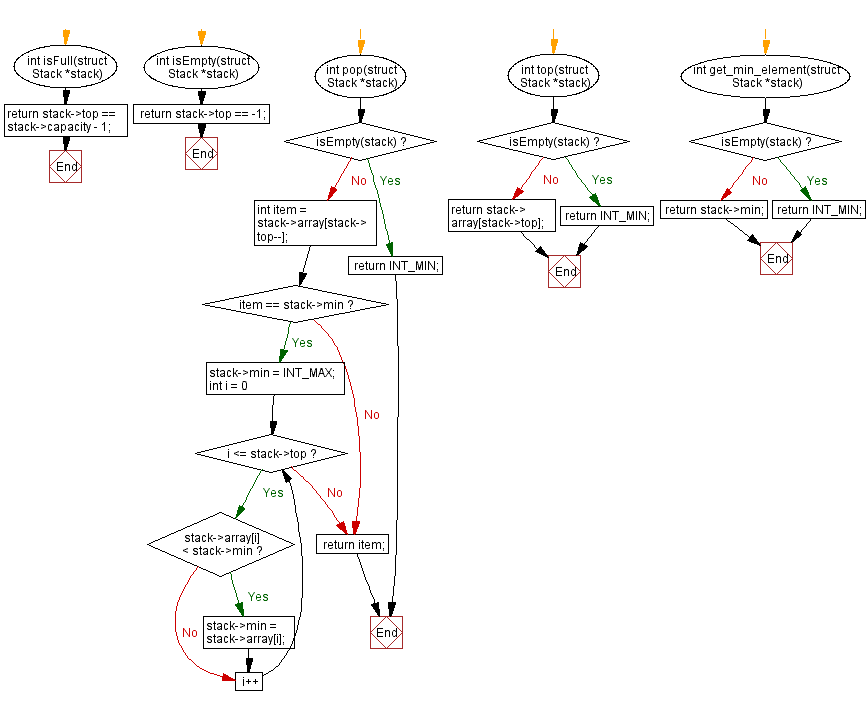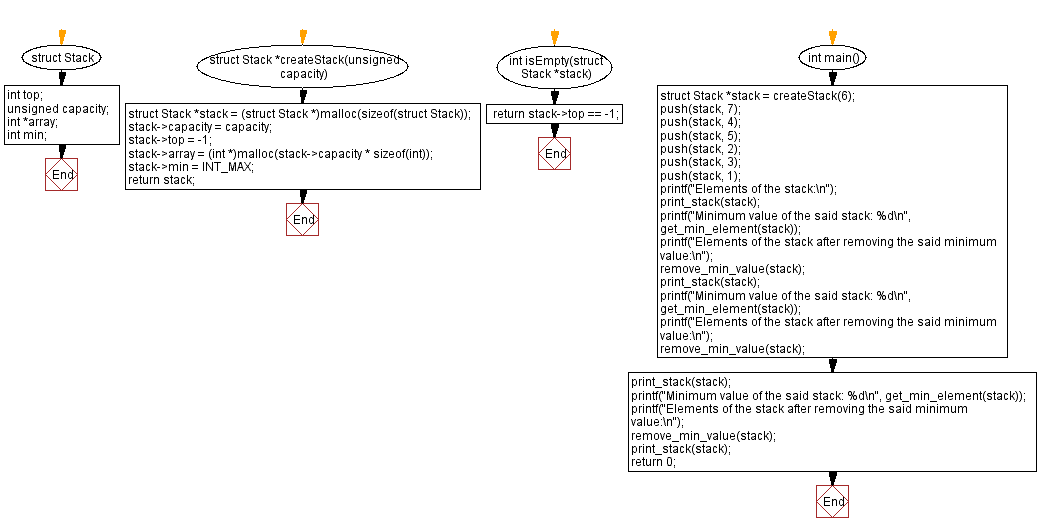﻿ C - Remove the minimum value from a stack

# C Exercises: Remove the minimum value from a stack

## C Stack: Exercise-15 with Solution

Write a C program to implement a stack and accept some numeric values. Remove the number whose value is the minimum on the stack.

Sample Solution:

C Code:

``````#include <stdio.h>
#include <stdlib.h>
#include <limits.h>

struct Stack {
int top;
unsigned capacity;
int *array;
int min;
};

struct Stack *createStack(unsigned capacity) {
struct Stack *stack = (struct Stack *)malloc(sizeof(struct Stack));
stack->capacity = capacity;
stack->top = -1;
stack->array = (int *)malloc(stack->capacity * sizeof(int));
stack->min = INT_MAX;
return stack;
}

int isFull(struct Stack *stack) {
return stack->top == stack->capacity - 1;
}

int isEmpty(struct Stack *stack) {
return stack->top == -1;
}

void push(struct Stack *stack, int item) {
if (isFull(stack)) {
return;
}
if (item < stack->min) {
stack->min = item;
}
stack->array[++stack->top] = item;
}

int pop(struct Stack *stack) {
if (isEmpty(stack)) {
return INT_MIN;
}
int item = stack->array[stack->top--];
if (item == stack->min) {
stack->min = INT_MAX;
for (int i = 0; i <= stack->top; i++) {
if (stack->array[i] < stack->min) {
stack->min = stack->array[i];
}
}
}
return item;
}

int top(struct Stack *stack) {
if (isEmpty(stack)) {
return INT_MIN;
}
return stack->array[stack->top];
}

int get_min_element(struct Stack *stack) {
if (isEmpty(stack)) {
return INT_MIN;
}
return stack->min;
}

void remove_min_value(struct Stack *stack) {
if (isEmpty(stack)) {
return;
}
int min = get_min_element(stack);
struct Stack *tempStack = createStack(stack->capacity);
while (!isEmpty(stack)) {
int item = pop(stack);
if (item != min) {
push(tempStack, item);
}
}
while (!isEmpty(tempStack)) {
push(stack, pop(tempStack));
}
}

void print_stack(struct Stack *stack) {
if (isEmpty(stack)) {
printf("Stack is empty\n");
return;
}
printf("Stack: ");
for (int i = 0; i <= stack->top; i++) {
printf("%d ", stack->array[i]);
}
printf("\n");
}

int main() {
struct Stack *stack = createStack(6);
push(stack, 7);
push(stack, 4);
push(stack, 5);
push(stack, 2);
push(stack, 3);
push(stack, 1);
printf("Elements of the stack:\n");
print_stack(stack);
printf("Minimum value of the said stack: %d\n", get_min_element(stack));
printf("Elements of the stack after removing the said minimum value:\n");
remove_min_value(stack);
print_stack(stack);
printf("Minimum value of the said stack: %d\n", get_min_element(stack));
printf("Elements of the stack after removing the said minimum value:\n");
remove_min_value(stack);
print_stack(stack);
printf("Minimum value of the said stack: %d\n", get_min_element(stack));
printf("Elements of the stack after removing the said minimum value:\n");
remove_min_value(stack);
print_stack(stack);
return 0;
}
```
```

Sample Output:

```Elements of the stack:
Stack: 7 4 5 2 3 1
Minimum value of the said stack: 1
Elements of the stack after removing the said minimum value:
Stack: 7 4 5 2 3
Minimum value of the said stack: 2
Elements of the stack after removing the said minimum value:
Stack: 7 4 5 3
Minimum value of the said stack: 3
Elements of the stack after removing the said minimum value:
Stack: 7 4 5
```

Flowchart:C Programming Code Editor:

Have another way to solve this solution? Contribute your code (and comments) through Disqus.

What is the difficulty level of this exercise?

Test your Programming skills with w3resource's quiz.

﻿

## C Programming: Tips of the Day

C Programming - How do you pass a function as a parameter in C?

Declaration

A prototype for a function which takes a function parameter looks like the following:

```void func ( void (*f)(int) );
```

This states that the parameter f will be a pointer to a function which has a void return type and which takes a single int parameter. The following function (print) is an example of a function which could be passed to func as a parameter because it is the proper type:

```void print ( int x ) {
printf("%d\n", x);
}
```

Function Call

When calling a function with a function parameter, the value passed must be a pointer to a function. Use the function's name (without parentheses) for this:

```func(print);
```

would call func, passing the print function to it.

Function Body

As with any parameter, func can now use the parameter's name in the function body to access the value of the parameter. Let's say that func will apply the function it is passed to the numbers 0-4. Consider, first, what the loop would look like to call print directly:

```for ( int ctr = 0 ; ctr < 5 ; ctr++ ) {
print(ctr);
}
```

Since func's parameter declaration says that f is the name for a pointer to the desired function, we recall first that if f is a pointer then *f is the thing that f points to (i.e. the function print in this case). As a result, just replace every occurrence of print in the loop above with *f:

```void func ( void (*f)(int) ) {
for ( int ctr = 0 ; ctr < 5 ; ctr++ ) {
(*f)(ctr);
}
}
```

Ref : https://bit.ly/3skw9Um